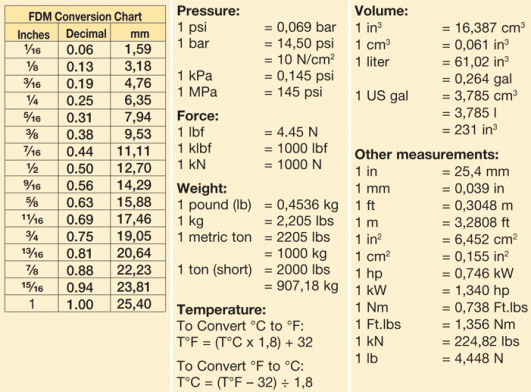# How Much Is 153 Cm's In Feet And Inches?

153cm equals 60.236 inches. There are 12 inches in a foot, so 60.236 inches would be 5 foot 2 inchesThe calculation to convert centimeters to feet and inches is as follows:

• Number of centimeters x 0.39 = amount in inches. Divide this number by twelve for feet and inches.
• Please note in this calculation, 0.39 has been rounded up to the nearest hundredth. For an absolutely accurate figure, use 0.3937008 instead of 0.39.

There are several online sites which can perform the calculation from imperial to metric (and vice-versa) instantly. You could try metric-conversions.org, thecalculatorsite.com or feettometres.com. As a general rule, remember 1 foot is roughly 0.3 of a meter.